# Working with Quadratic Functions in Trigonometry: Tutoring Solution Chapter Exam

Exam Instructions:

Choose your answers to the questions and click 'Next' to see the next set of questions. You can skip questions if you would like and come back to them later with the yellow "Go To First Skipped Question" button. When you have completed the practice exam, a green submit button will appear. Click it to see your results. Good luck!

### Page 1

#### Question 3 3. Find the value for c and rewrite the equation in the vertex form.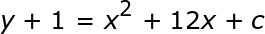### Page 2

#### Question 6 6. How many roots does the equation below have?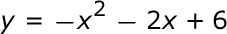#### Question 9 9. Expressing the equation below in the vertex form will give a value of 'h' = _____ .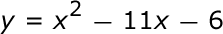### Page 3

#### Question 12 12. When completing the square on the quadratic equation listed below, how is c calculated?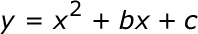#### Question 13 13. If the equation below told you a ball was 'h' feet in the air after 't' seconds, what would be the first step to finding out when it hit the ground?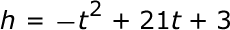### Page 4

#### Question 18 18. What would the first step be in completing the square of the following equation?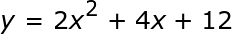#### Question 19 19. Find the roots of the equation below: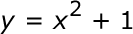#### Question 20 20. Which statement MUST be true about the equation below?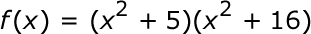### Page 5

#### Question 24 24. Rewrite the following equation in the vertex form.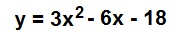### Page 6

#### Question 26 26. Solve the equation below.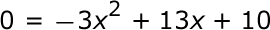#### Question 27 27. What inequality symbol is represented by the red dashed line?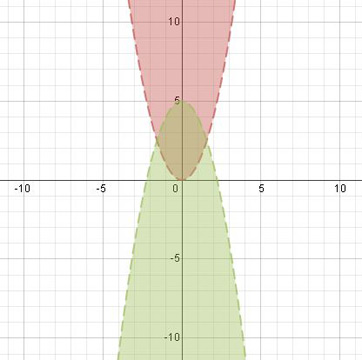#### Question 28 28. What is the most simplified form of the expression below?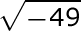#### Question 29 29. In order to complete the square on the following equation, what value would you add to both sides?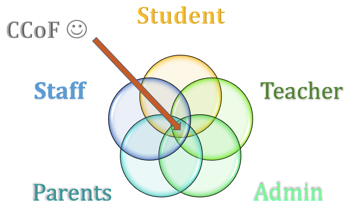#### Question 30 30. Solve the equation below.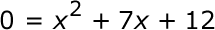#### Working with Quadratic Functions in Trigonometry: Tutoring Solution Chapter Exam Instructions

Choose your answers to the questions and click 'Next' to see the next set of questions. You can skip questions if you would like and come back to them later with the yellow "Go To First Skipped Question" button. When you have completed the practice exam, a green submit button will appear. Click it to see your results. Good luck!

Support